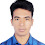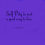# Slab design calculation one way and two way method in excel sheet with example

##Civil or structural engineer know that how to calculated slab design. Here you can get a free excel sheet where a engineer easily design one way or two way slab by one click. We also explain a slab design example in this excel sheet.

# Slab design calculation one way and two way method:

#### An Example of two way design calculation:

Input data:

Slab Dimensions:

Short span = 10 ft.
Long Span = 15 ft.
Span ratio = 10/15 = 0.67
Slab thickness (reqd) = 2 x (10+15) x 12/180 = 3.33 in
Slab thickness (prvd) = 5 in.

Edge Conditions:

Short span edge condition = Both edges continuous
Long span edge condition = Both edges discontinuous

Slab self wt = 62.50 psf.
Service live load = 75 psf.
Material Strengths:
Concrete Strength = 2.5 ksi.
Steel yield strength = 60 ksi.

Design Moments

Total ultimate DL = 1.4x(62.5+40) = 143.5 psf.
Total ultimate LL = 1.7x(75) = 127.5 psf.
Total ultimate load = 143.5+ 127.5 = 271 psf.

Short Span Moments:

Positive Moment DL Muall(dl)+ = 0.036 x 143.5 x 10^2 = 0.517 k-ft.
Positive Moment LL Muall+ = 0.054 x 127.5 x 10^2 = 0.689 k-ft.
Total positive short span moment mua+ = 1.205 k-ft.
Negative Moment on Discontinuous Edge = 1/3 x 0.416 = 0.139 k-ft.
Negative Moment on Discontinuous Edge = 1/3 x 0.416 = 0.139 k-ft.

Reinforcement Calculations

Shear Check:
Total load on the panel = 10 x 15 x 288.5 = 43.2 kips
Shear Capacity of the section fVc = 0.85 x 2 x ( 2500 )^½ x 12 x 5 / 1000
= 5.1 k/ft
Shear along short side      Vua  =  ( 0.04 x 43.2 ) / (2 x 10 )
= 0.08 k/ft    <5.1 k/ft…. (O.K.)
Shear along short side      Vub =( 0.04 x 43.2 ) / (2 x 10 )
= 0.08 k/ft <5.1 k/ft…. (O.K.)
Short Span Moments:
Positive Moment DL Mua(dl)+ = 0.036 x 161 x 10^2  = 0.580 k-ft
Positive Moment LL Muall+ = 0.054 x 127.5 x 10^2 = 0.689 k-ft
Total Postive Short Span Moment Mua+ =  1.268 k-ft
Negative Moment on Continuous Edge = 0.087 x 288.5 x 10^2  = 2.510  k-ft
0.087 x 1.268
0.087 x 288.5 x 10^2
Negative Moment on Continuous Edge = 0.087 x 288.5 x 10^2 = 2.510 k-ft
0.087 x 1.268
0.087 x 288.5 x 10^2
Long Span Moments:
Positive Moment DL Mubdl+ = 0.004 x 161 x 15^2  = 0.145 k-ft
Positive Moment LL Mubll+ = 0.01 x 127.5 x 15^2  = 0.287 k-ft
Total Postive Long Span Moment Mub+  = 0.432  k-ft
Negative Moment on Discontinuous Edge = 1/3 x 0.432 = 0.144 k-ft
1/3 x 0.432
1/3 x 288.5 x 15^2
Negative Moment on Discontinuous Edge = 1/3 x 0.432 = 0.144 k-ft
1/3 x 0.432
1/3 x 288.5 x 15^2

If you insert One way slab data. then this calculation result show for one way slab.

For any problem in this design calculation excel sheet, comment blew, thank you.

1.Thanks.

1.2.3.4.5.